# Creating a Function

Functions are defined in JavaScript using the following syntax:

` function myFunc (parameter1,...,parametern) {    statement1;    ...    statementn;    return retval; //specify optional return value  } `

In plain English, the following are the steps involved in creating a function.

To Create a Function:

2. Provide the identifier (or name) that will be used for the function.

3. Within parentheses, provide a list of names for the parameters (also called arguments) that will be passed to the function. The parameter names are separated by commas. If there are no parameters, just use empty open and close parentheses, like so: ().

4. Place the code statements that will comprise the function within curly braces. Here are some curly braces: { }.

5. If the function returns a value to the code that called it, use the return keyword within the function statements to specify the return value. Note that when the return keyword is used, program execution returns to the code that invoked the function—so any statements in the function following the return statement will not be processed.

You invoke (or call) a function by using the function as a statement or assignment. Here are a number of examples:

` myFunc();  x = myFunc2();  myFunc3 (par1, par2, par3);  result = myFunc4 (par1, par2, par3); `Try This at Home—Functions and Children First!

You should know that if you’re placing JavaScript code in a Web page (as in the examples in this book), you need to define a function before it can be used. Web pages are processed by the browser from the top down, so a function must be located above the code that calls the function in the Web page. This isn’t the case in many programming environments. It’s far more typical to be able to place your functions anywhere in your program that you’d like.

One way to make sure that a function has been processed before the code that calls the function is encountered is to place your functions in an HTML document head section rather than in the body of the HTML document.The first of these statements, myFunc();, calls the function named myFunc, which neither takes nor returns any values.

The next statement, x = myFunc2();, calls the function myFunc2, which doesn’t accept a passed value but does return a value. The returned value of the function myFunc2 is then assigned to the variable x.

The statement myFunc3 (par1, par2, par3); calls the function myFunc3 with three passed values, but no value is returned. (The values passed to a function are often called arguments or parameters.)

The final statement, result = myFunc4 (par1, par2, par3);, calls the function myFunc4 with three arguments. The function returns a value, which is assigned to the variable result.

I think you probably get the idea of how functions work and how to call them! But, as always, it’s easiest to see how functions work in practice. So it’s time to create a simple example.

### The Only Good Return Is a Hard Return

The example I’ll show you for creating a function, and then calling it, is pretty simple. But it actually has some practical use.

A lot of times, when you display text, you’d like to split it up on more than one line. Lines of text are broken up for printing or display using a hard return. In the HTML used to create Web pages, a hard return is created using the <br> tag.

For example, the following HTML fragment:

` <HTML>     This is one line; <br>     this is another line; <br>     and this is the final line  </HTML> `

uses a hard return generated with the <br> tag to display text on three lines, as shown in Figure 5-1.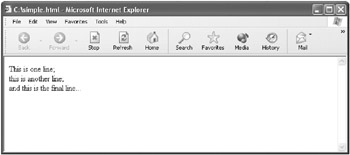Figure 5-1: In an HTML document, the <br> tag is used to create a line break.

If you know you’re going to be inserting line breaks in your text, wouldn’t it be nice not to have to type all those <br> tags? It’s easy enough to write a JavaScript function that extends the text display generated by the JavaScript document.write method, adding a <br> tag to the end of the text to be displayed. This kind of extension of an existing function or method is sometimes called wrapping —because the inner function is wrapped in another function.

 Note To wrap a function or method means to call it from within another function. The wrapping function generally adds some functionality to the wrapped function.

We’ll call the function that adds a line break to the HTML text displayed: writeMyWay.

To Create a Function That Adds a Line Break to Displayed Text:

1. In an HTML document, above the place you want to use the function (possibly in the <HEAD> section of the document), start by entering the keyword function.

2. Name the function (writeMyWay).

3. Provide an input parameter (textIn) so that the function knows it’ll be passed a value.

4. Enter an opening and closing curly brace: {...}. In many languages, including JavaScript, it’s conventional (but not required) to skip some lines between the curly braces—that’s where the actual work of the function is accomplished.

So far what we’ve entered is called the declaration of the function, and it looks like this:

` function writeMyWay (textIn) {  } `

5. Next, within the curly braces, add the code that makes the function work. This code calls the JavaScript document.write method, using the text passed into the function with a line break appended to the text. Here’s the writeMyWay function including the code that displays the text with an added line break:

` function writeMyWay (textIn) {     document.write (textIn + "<br>");  } `

Note that the writeMyWay function doesn’t return a value to the call code—it just displays text.

You’ll find the function in an HTML page that also invokes it in Listing 5-1.

Listing 5.1: The Function That Wraps Document.Write, Adding a Line Break` <HTML> <BODY> <CENTER> <H1> <SCRIPT>     function writeMyWay (textIn) {        document.write (textIn + "<br>");     }     <!-- Call the writeMyWay function-->     writeMyWay ("I");     writeMyWay ("did");     writeMyWay ("it");     writeMyWay ("my");     writeMyWay ("way!");     </SCRIPT> </CENTER> </H1> </BODY> </HTML> `The next thing to do is to create an example that calls the function. This is easy enough!

To Call the writeMyWay Function:

1. Within an HTML document, after the function has been defined, call the writeMyWay function with the text you want displayed on its own line:

` writeMyWay ("I");  writeMyWay ("did");  writeMyWay ("it");  writeMyWay ("my");  writeMyWay ("way!"); `

2. Open the HTML document in a Web browser. Each bit of text will be displayed on its own line, as you can see in Figure 5-2.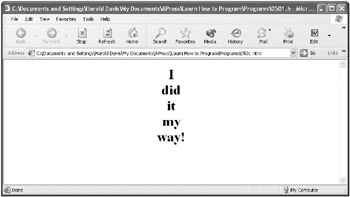Figure 5-2: The function adds a line break to the text passed to it.

Listing 5-1 shows the writeMyWay function and the code for calling it.

This seems (and is) easy enough. Let’s have a look at another example in which the function returns a value.

### Returns Are Good

Let’s have a look at another simple function, one that returns as a value the sum of three numbers. Obviously, there’s no great intellectual feat in adding three numbers together. But this example shows you how to return a value from a function (and this works the same way even if the function is a great deal more complicated). In addition, the example allows you to start having a look at two other important topics:

• Types and type conversion

• Creating an HTML form to use JavaScript code to allow interactivity

As I explained earlier in this chapter, to return a value from a function, you simply use the return keyword and specify the value to be returned. (When a function is processed, execution of code statements within the function stops after the value is returned, and execution control returns to the code that called the function.)

So you’d think it would be a simple enough matter to write a function that returns the sum of three numbers. Except for one wrinkle, it is!

Let’s start with a function that accepts three inputs as passed values (or arguments):

` function addThreeNums (inOne, inTwo, inThree) {  } `

As in normal, everyday arithmetic, the plus sign (+), called the plus operator, is used to sum (add) numbers. So, logically, it’s easy to add the three input numbers:

` inOne + inTwo + inThree `

If you add the return keyword, for a first attempt, the function that adds three numbers and returns the sum would look like this:

` function addThreeNums (inOne, inTwo, inThree) {     return inOne + inTwo + inThree;  } `

This looks pretty good, but as I mentioned, there’s a wrinkle (which is another way of saying that there’s a problem with this code).

If the computer thinks that the inputs to this function are text strings rather than numbers, it’ll also think that the plus sign is used for string concatenation rather than numeric addition.

Here’s an example of string concatenation: Let’s suppose you have three text strings: I, love, and you. You can then use the string concatenation operator like this:

` var kissyFace = "I" + "love" + "you"; `

The string value stored in the variable kissyFace is now "I love you".

Returning to our function, let’s suppose that the computer thinks that the plus sign means that string concatenation (rather than numeric addition) is taking place. In this case, have a look at the function’s return statement:

` return inOne + inTwo + inThree; `

Suppose the value stored in the variable inOne is 1, the value stored in inTwo is 2, and the value stored in inThree is 3. If these values are concatenated together, the result returned will be 123. This is a pretty far cry from adding the values, which returns a result of 6.

It’s important to understand that a value stored in a variable can appear to be numeric and still be a string. In other words, as an example, 2 can be either the number 2 or the string "2". The computer decides that the + operator means to concatenate rather than to add if at least one of the values being operated on is a string.

So how does the computer decide whether a value is numeric or a text string? When a loosely typed computer language such as JavaScript is used, basically the computer makes a gut assessment depending on the context of the value. Like many human gut assessments, or best guesses, this can often be wrong!

As I’ve already said, if the computer thinks that the values passed to the addThreeNums function are strings—such as the string "2" rather than the numeric value 2—the function will produce the wrong result. Specifically, it’ll concatenate the inputs rather than adding them.

The worst part of it is that the way this function is likely to be used in the real world is that much of the time the values passed to it will be taken for strings rather than numbers. In the example I’m about to show you, the user inputs three numbers. Then the addThreeNums function is called to sum the user’s input and display the results. The problem is that by definition in JavaScript a user input is a string, even when it’s a number.

How can we correct this problem?

The answer is that we can explicitly convert each input to the addThreeNums function to a number using the Number function built into JavaScript. Here’s what the revised function looks like:

` function addThreeNums (inOne, inTwo, inThree) {     var inOne = Number(inOne);     var inTwo = Number(inTwo);     var inThree = Number(inThree);     return Number(inOne + inTwo + inThree);  } `Advanced—Understanding Loosely and Strictly Typed Computer Languages

Computer languages can either be loosely typed, such as JavaScript, or strictly typed (such as Java and C#). (Some other languages, such as Visual Basic .NET, are actually hybrids, and can be used in either a loosely typed mode or a strictly typed mode.)

In a strictly typed language, as opposed to a loosely typed language, the programmer must specify the type of each variable that will be used to store values. In the context of a programming language variable, a type isn’t an animal, vegetable, or mineral—but rather refers to the types available in the programming language. In most modern programming languages, primitive types such as integer number, floating-point number, and text string are available. (I say primitive types because in modern programming languages it’s also generally possible to define your own types.)

In addition to specifying the types of all variables, in a strictly typed programming language the programmer is responsible for specifying methods for converting values from one type to another, at least if there’s any possibility of losing data in the conversion. In other words, for the most part, type conversions won’t take place automatically.

In contrast, in loosely typed languages you don’t have to specify the type of a variable. (In fact, in JavaScript you can’t specify a variable’s type when the variable is declared even if you wanted to specify it.) The computer does the best job it can figuring out the variable type based on the value assigned to the variable. Conversions take place automatically when the computer figures it’s appropriate, using the computer’s best guess for the conversion method to use.

In favor of loosely typed languages, they’re easier to use and require less up-front work. For better (and worse), strictly typed languages require programmers to be more precise. This means that programmers have to think a little harder about the types of values that will be used in their programs. The up-front work of understanding the types of the values that will be used in the program and specifying the conversion methods to be used generally leads to fewer errors in the finished code.Each of the values passed to the function, as represented by a function parameter such as inOne, are converted to a new variable (with the same name, for example, inOne) using the Number function:

` var inOne = Number(inOne); `

Then the three inputs are added, and the resulting value is returned:

` return Number(inOne + inTwo + inThree); `

A few comments are worth making. If the inputs to the function are already of numeric type, then calling the Number function has no effect— which is just fine!

But what happens if one of the inputs to the function is something that can’t be converted to a number, for example, the text string Harold? Good question! In that case, the results of the conversion using the Number function are a JavaScript value of NaN, which is short for Not a Number. In fact, if you add a value of NaN to numbers, you still get NaN. So, if our addThreeNums function tries to add the text string Harold to 1 and 2, the result will be NaN.

It’s a good criticism of the example I’m about to show you that there’s no testing to make sure that only values that can be converted to numbers are sent to the addThreeNums function. It’s actually pretty easy to make this kind of test—called validating user input —by first attempting to convert the value input to a number and then checking to see that result isn’t NaN. In fact, if you wanted, you could “bullet proof” the addThreeNums function by adding a test of this sort for each input to the function before attempting to add the inputs.

The Number conversion function is closely related to the JavaScript Number object. You’ll learn more about objects in JavaScript in Chapter 7, “ Working with Objects.”

 Note By the way, sometimes you’ll need to explicitly force a conversion of a number to a text string—in other words, convert in the opposite direction from the Number function. You do this using the String function built into JavaScript.

Getting back to our function, and our example, it’s time to construct an HTML form that will allow the user to enter three numbers. When the user clicks the Add Them button, the AddThreeNums function adds the numbers. Finally, the results are displayed using the document.write method.

To Allow User Input of Three Numbers and Display The Sum:

1. Place the addThreeNums function in the <HEAD> section of an HTML document:

` <HTML> <HEAD> <SCRIPT>     function addThreeNums (inOne, inTwo, inThree) {        var inOne = Number(inOne);        var inTwo = Number(inTwo);        var inThree = Number(inThree);        return Number(inOne + inTwo + inThree);     }     </SCRIPT> </HEAD> <BODY>  ...  </BODY> </HTML> `

2. In the <BODY> section of the HTML document, add an HTML form:

` <BODY> <FORM>     ...     </FORM> <BODY> `

3. So that the elements of the HTML form can be referred to in JavaScript code, use a Name attribute to name the form:

` <BODY> <FORM Name="theForm">     ...     </FORM> <BODY> `

4. Within the HTML form, add three text input elements, one for each of the numbers to be input, and name each element:

` <INPUT Type=Text Name="num1"> <INPUT Type=Text Name="num2"> <INPUT Type=Text Name="num3"> `

5. Within the HTML form, add a button element:

` <INPUT Type=Button> `

6. Provide a Value attribute for the button (this is the text that will appear on the button in the browser):

` <INPUT Type=Button Value="Add Them"> `

7. Add an onClick handler to the button:

` <INPUT Type=Button Value="Add Them" onClick= > `

8. The JavaScript code placed within quotes and assigned to the onClick handler is executed when the button is clicked by the user. Single quotes are used so that double quotes can be employed within the JavaScript code. (You’ll find more about JavaScript events, such as the onClick event in Chapter 8, “ Understanding Events and EventDriven Code ”). Here’s the code assigned to the onClick event that calls the addThreeNums function with the values entered by the user and uses the document.write method to display the results:

` <INPUT Type=Button Value="Add Them"  onClick='document.write("The sum of the three numbers is " +  addThreeNums(theForm.num1.value, iconid=parrow     theForm.num2.value, theForm.num3.value));'> `

9. Save the HTML file (Listing 5-2 shows the complete code).

Listing 5.2: A Function That Returns the Sum of Three Numbers (Stripped-Down Version)` <HTML> <HEAD> <TITLE>     Add three numbers     </TITLE> <SCRIPT>     function addThreeNums (inOne, inTwo, inThree) {        var inOne = Number(inOne);        var inTwo = Number(inTwo);        var inThree = Number(inThree);        return Number(inOne + inTwo + inThree);     }     </SCRIPT> </HEAD> <BODY> <FORM Name="theForm"> <INPUT Type=Text Name="num1"> <INPUT Type=Text Name="num2"> <INPUT Type=Text Name="num3"> <INPUT Type=Button Value="Add Them"           onClick='document.write("The sum of the three numbers is " +  addThreeNums(theForm.num1.value,theForm.num2.value,theForm.num3.value));'> </FORM> </BODY> </HTML> `10. Open it in a Web browser to test the function.

11. Enter a number in each of the text boxes (see Figure 5-3).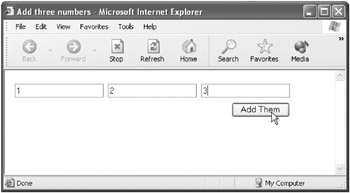Figure 5-3: The user can enter a number in each of the text boxes.

12. Click Add Them. The document.write method is used to display the sum of the three numbers (see Figure 5-4).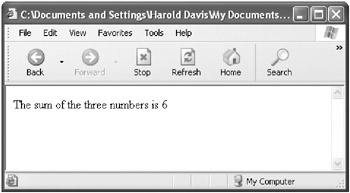Figure 5-4: The sum of the three numbers is displayed.Learning More About HTML Table Tags

You don’t really need to use the HTML shown in Listing 5-3 to get the programming point of the exercise. And I can’t really explain HTML (which isn’t programming anyhow) within the scope of this book. (There are quite a few good books just about HTML that you can get.) But let me quickly explain some of the table tags shown in Listing 5-3:

• <TR> </TR> tags mark a row in a table.

• <TD> </TD> tags mark a cell within a row in a table.

• The cellspacing attribute of the <TABLE> tag determines the number of pixels separating each table cell.

Listing 5.3: The Function That Returns the Sum of Three Numbers Including Table Codes` <HTML> <HEAD> <TITLE>     Add three numbers     </TITLE> <SCRIPT>     function addThreeNums (inOne, inTwo, inThree) {        var inOne = Number(inOne);        var inTwo = Number(inTwo);        var inThree = Number(inThree);        return Number(inOne + inTwo + inThree);     }     </SCRIPT> </HEAD> <BODY> <FORM Name="theForm"> <TABLE cellspacing=5> <TR> <TD> <INPUT Type=Text Name="num1"> </TD> <TD> <INPUT Type=Text              Name="num2"> </TD> <TD> <INPUT Type=Text Name="num3"> </TD> </TR> <TR> <TD> </TD> <TD> </TD> <TD align=right> <INPUT Type=Button Value="Add  Them"           onClick='document.write("The sum of the three numbers is " +         addThreeNums(theForm.num1.value, theForm.num2.value,  theForm.num3.value));'> </TD> </TR> </TABLE> </FORM> </BODY> </HTML> `There are some “gotchas” with this application. First, as I’ve already mentioned, no validation of user input is performed to make sure that the user has entered something that can actually be converted to a number (this validation check could be performed when the user enters a value or in the function itself).

Second, it’s of course no big shakes to add three numbers. The important point is that this example shows how to return a value from a function.

Finally, I lied to you. What, an author who lies? Never! The HTML shown in Listing 5-2 would never look as clean and orderly as the user interface shown in Figure 5-3. As experienced creators of HTML documents understand, HTML tables are generally used to organize screens in HTML applications. This is a book about learning the craft of programming, not about creating HTML documents. So I thought it was a good idea in Listing 5-2 to show the HTML form and JavaScript code it invokes without complicating matters by showing the HTML table tags used for formatting purposes. But, if you want to see the HTML form, JavaScript code, and HTML table tags used for formatting, look at Listing 5-3.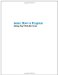Learn How to Program Using Any Web Browser
ISBN: 1590591135
EAN: 2147483647
Year: 2006
Pages: 115
Authors: Harold Davis

Similar book on Amazon